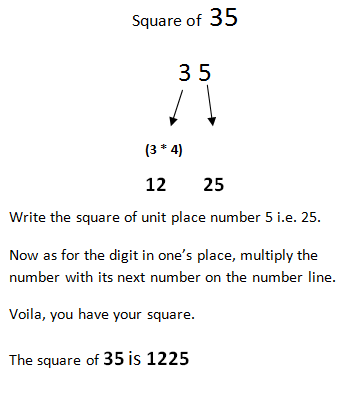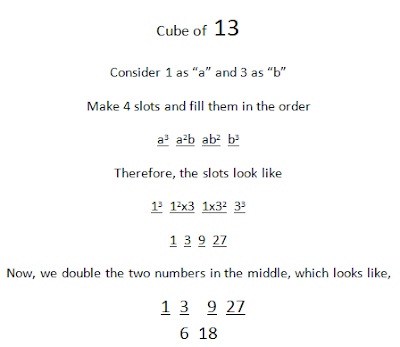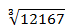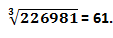# Shortcut Tricks of Square & Cubes For Bank Exams### To find the square of a two-digit number,Easy enough!

Couple points to remember
In the above example, the square of 3 is written as 09. This is JUST applicable for numbers 1, 2 and 3 as the squares of these numbers are in single digits. For further numbers i.e. 4, 5, 6 & so on, the squares used in the calculation will be the square itself. E.g.• The number 20 while calculation remains constant.
• Having written the squares individually, consider them as a whole. E.g. 916 in the above example.
• This method can also help you in finding the squares of 3-digit numbers but the pair should be considered as
• If the number is 108. The numbers to be considered in calculation will be
• 10 8
• To find the square of two-digit number with the unit place 5.This is all about squares; let us now switch to Cubes,
To find the cube of 2-digit numberNow, we add. To add we just take down the unit digit, leaving everything else for the carry, which looks like,Taking the number 18, as next example,Now, we add. To add we just take down the unit digit, leaving everything else for the carry, which looks like,

Let us now move to square roots and cube roots.

Before starting the calculation for roots, you need to memorize squares and cubes along with roots from 1 to 10. This will help make the calculation fast.To find the square root of a number

Consider a number. √529
To find the square root of a number, we start by making pairs in the number from right hand side, which looks like            5   29
• Having done that, we work from the unit digits. As we can see the unit digit in the second pair is “9”. After looking at the squares column in the above table, we can say that there are two numbers “3” and “7” with squares having unit digit 9. Therefore, one thing we can say with certainty that the unit digit of the square root of “529” will be either 3 or 7.
• That is all we needed the pair 29 for.
• Now, to find the digit preceding 3 or 7, we need to work on the second pair, which in this case is “5”. Consulting the square column in the above table, we can say that number 5 lies between “4” and “9” which are the respective squares of “2” and “3”.
• We consider the smallest square root among the two which is “2” followed by the number “3” or “7”, from the last conclusion.
• This reduces the square root calculation to just the two numbers which are now either 23 or 27.
• Now, we need a reference of comparison between the two numbers and there is a number between 23 and 27 whose square for us to determine is a cake-walk which is the number with the unit digit 5, In this case “25”. So, we find the square of 25 in no-time, which is “625” (Using the trick mentioned above).
• Furthermore, the number we were provided to find the square root of was “529”, which is smaller than 625, thus making the root of 529 less than that of 625. From the two numbers that we predicted i.e. 23 and 27, the number smaller than 25 is 23, thus confirming the prediction and the process.
Therefore, the square root of 529 is 23.

### Concluding the process into steps:

1. We divide the given numbers into two pairs starting from the right-hand side. We take the last two numbers and mark it pair no. 1 while the remaining ones form pair no. 2.

2. After that we match the unit digit of the pair no. 1 i.e. unit digit of the number with the squares of the natural numbers ranging from 1 to 10. We get two numbers having the same unit digit. These two digits form the unit digit of our final square root.

3. Having done this, the significance of the pair no. 1 expires, now we need to work on the other pair. We work on the pair by matching it with the squares of the natural numbers. We take the square root when the number is matched. If not, the number must lie between any two squares, the smaller square root among the two is considered.

4. We combine the digit we get from the two processes. The number from pair no. 1 gives the unit digit while the number from pair no. 2 gives the remaining number.

5. We still have two numbers and need to make a decision. For the reference of comparison, we take a number between these two numbers having a unit digit 5(because the process of finding the squares of number with unit digit 5 is comparatively easy). We compare the square of number with unit digit 5 to the number provided to us.

6. If the number given to us is smaller, the square root will be smaller than the number with unit digit 5 or vice-versa, thus providing us with one single number in the end which forms the square root.

7. Not to worry coz the two numbers we predict at the end of step no. 4, one of them is smaller while the other is always greater than the number with unit digit 5.

Let’s take one more example, making the process crystal for you guys,

### Question 1.

Find √1444

Solution: Step- to-step compilation
14 44
Based on the unit digit which is 4 and there are two numbers from 1 to 10 having squares with unit digit 4 i.e. 2 (square 4) and 8(square 64). Therefore, the unit digit of the number is either 2 or 8.We need a reference of comparison between 32 and 38 and 35 gives us an easy option.As, we can see the number 1444 is greater than 1225, therefore, the square root must be larger than 35 too. And among 32 and 38, the number larger than 35 is 38(making it the final answer).Bit of a practice and you will get a hold of it.

Now, moving on to the process of finding cube root,

To find the cube root of a number,The process is similar to the one we did in the square root.

To begin with, we group the numbers starting from the right hand side i.e. unit digit of the number, similar to what we did in squares. Only difference is that we used last two digits to make the pair, in this case we use last three digits to make the group.

12 167

Now, we use last three digits to determine the unit digit.

Matching the unit digit in the given number to the cubes column in the above table, we see that 27 is the number with the same unit digit as 167. We take the cube root of 27 which is 3 and it forms the unit digit of our predicted square root.

The process of working on the next group is similar as it used to be in the square root. The number 12 lies between “8” and “27” in the cube root column. “2” and “3” are the cube roots respectively.

We consider the smaller cube root and place it before the predicted unit digit.

The number thus formed is 23(final answer).Not to worry, we take another example.
226   981
Based on the unit digit of the last three numbers of the given number which is 981, we check the cubes column and the number with the unit digit similar to 981 is 1. We consider the cube root of 1, which is again 1, thus making the unit digit of our prediction 1.To work with the second group, the number 226 in the cubes column lies between 216 and 343 which are the cubes for 6 and 7 respectively.We consider the smaller number which is 6 and placing the prediction of the unit digit after it. The number formed is 61(final answer).Little bit of practice will help save some precious seconds in the exam over the traditional methods. Hope this tricks help.#### What's trending in BankExamsToday

Smart Prep Kit for Banking Exams by Ramandeep Singh - Download here